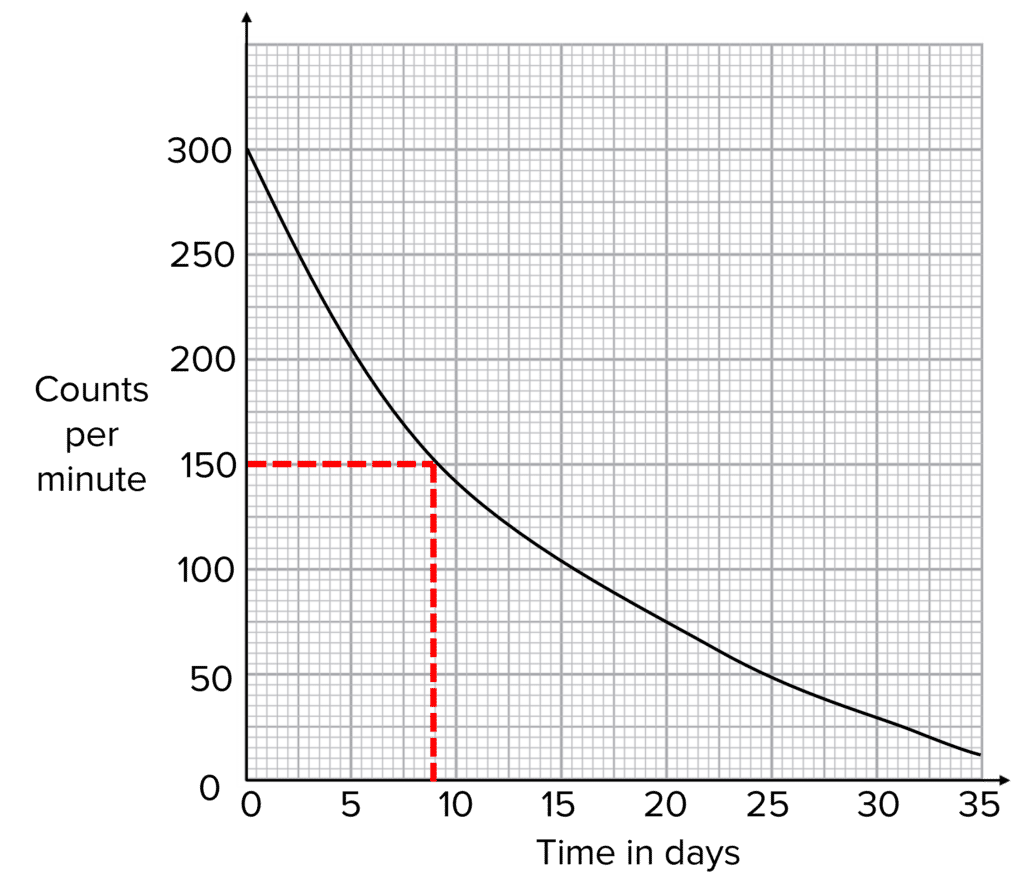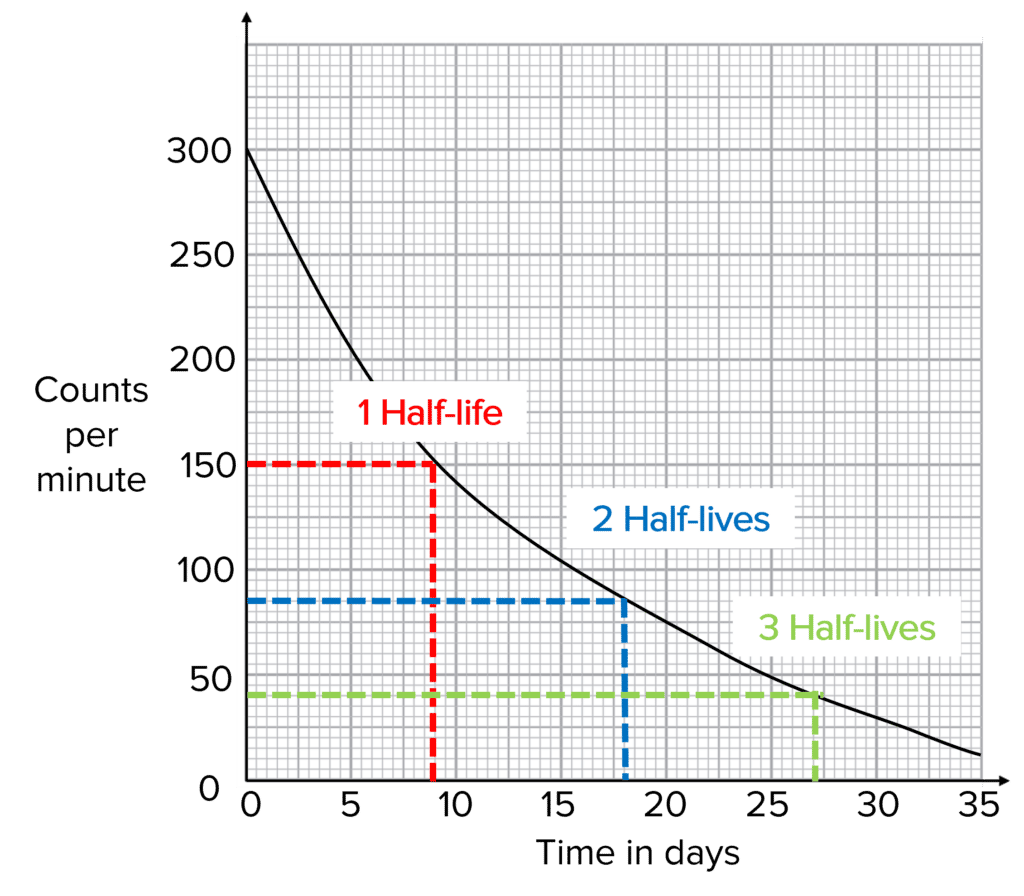GCSEAQACombined Science FoundationCombined Science HigherPhysics FoundationPhysics Higher

Because radioactive decay is random, you cannot predict when a single nucleus will decay. However, you can predict how long it will take for half of the nuclei in a sample to decay. This is called the half-life of the sample.

## Calculating Half-lifeThe definition of half-life is the time taken for the count rate from a sample to decrease to half the initial value. If we use a radiation detector, such as a Geiger-Muller tube, we can measure the radiation being emitted from a a sample and calculate the radioactive half life from the results.

First, you need to plot a graph of the counts per minute against the time. Then, extrapolate the time taken for the counts to reduce by a half. In this example, the original counts was measured to be $300 \text{ counts per minute}$ and so half the counts is $150 \text{ counts per minute}$. You can see from the graph that this corresponds to $9 \text{ days}$. Therefore the half-life of this sample is $9\text{ days}$

GCSECombined Science FoundationCombined Science HigherPhysics FoundationPhysics HigherAQA

## Calculations Using Half-LifeIf we know the half-life of a sample we can determine how much smaller the count rate will be after a given number of half-lives.

For example, after $2\text{ half-lives}$, the radioactivity of a sample will be $\dfrac{1}{2}\times \dfrac{1}{2} = \bold{\color{f21cc2}{\dfrac{1}{4}}}$ of the original radioactivity. This is the same as saying it is $4$ times smaller.

After $3\text{ half-lives}$, the radioactivity will be $\dfrac{1}{2} \times \dfrac{1}{2} \times \dfrac{1}{2} = \bold{\color{f21cc2}{\dfrac{1}{8}}}$ of the original activity, or $8$ times smaller.

GCSECombined Science HigherPhysics HigherAQA

The time taken for the radioactivity of a sample to reduce by halfGold Standard Education

You cannot predict when an individual nucleus will decayGold Standard Education

Half of the original counts per minute is $\dfrac{140}{2}=\bold{70}$

(Using graph) half-life is therefore $\bold{1.4}\text{ minutes}$ (or any value between $1\text{ minutes}$ and $1.5 \text{ minutes}$).Gold Standard Education

$4 \text{ hours} = \bold{4\text{ half-lives}}$

$100\text{ Bq} \times \dfrac{1}{2} \times \dfrac{1}{2} \times \dfrac{1}{2} \times \dfrac{1}{2} = \bold{6.25\text{ Bq}}$